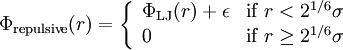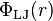# Weeks-Chandler-Andersen reference system modelThis article is a 'stub' page, it has no, or next to no, content. It is here at the moment to help form part of the structure of SklogWiki. If you add sufficient material to this article then please remove the {{Stub-general}} template from this page.

The Weeks-Chandler-Andersen reference system model consists of the purely repulsive part of the Weeks-Chandler-Andersen perturbation theory, i.e.$\Phi_{\rm repulsive} (r) = \left\{ \begin{array}{ll} \Phi_{\rm LJ}(r) + \epsilon & {\rm if} \; r < 2^{1/6}\sigma \\ 0 & {\rm if} \; r \ge 2^{1/6}\sigma \end{array} \right.$

where$\Phi_{\rm LJ}(r)$ represents the Lennard-Jones model.

## Colloids

Has been used as a model for colloids .